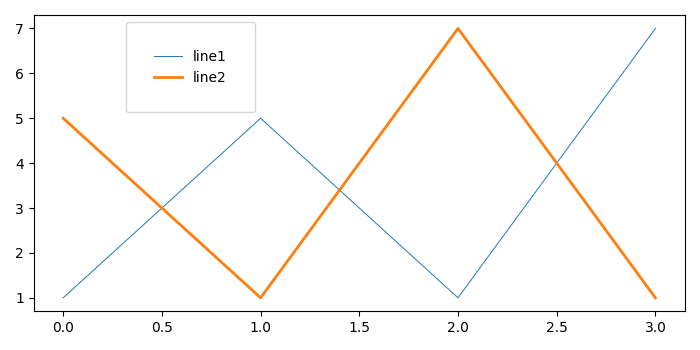# How to adjust the size of a Matplotlib legend box?

To adjust the size of a matplotlib legend box, we can use borderpad arguments in the legend method.

## Steps

• Create line1 and line2 using two lists with different line widths.

• To place a legend on the figure and to adjust the size of legend box, use borderpad=2 in legend() method.

• To display the figure, use show() method.

## Example

from matplotlib import pyplot as plt
plt.rcParams["figure.figsize"] = [7.00, 3.50]
plt.rcParams["figure.autolayout"] = True
line1, = plt.plot([1, 5, 1, 7], linewidth=0.7)
line2, = plt.plot([5, 1, 7, 1], linewidth=2.0)
plt.legend([line1, line2], ["line1", "line2"], bbox_to_anchor=(0.35, 1.0), borderpad=2)
plt.show()

## Output